# Positive Feedback

The use of positive feedback is useful for producing oscillators. The condition for positive feedback is that a portion of the output is combined in phase with the input. For an amplifer with positive feedback the gain is given by the expression below.

 The large open loop gain of an op-amp makes it inevitable that the condition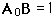will be reached, and the gain expression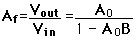becomes infinite.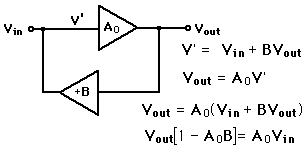Practically speaking, the gain which applies at low signal amplitudes will be reduced until the output amplitude reaches some constant value. However, that limiting value will be independent of input, allowing the circuit to produce a designed output.

Index

Electronics concepts

Op-amp concepts

Feedback concepts

 HyperPhysics*****Electricity and magnetism R Nave
Go Back

# Op-Amp Oscillators

 Phase-Shift Oscillator Colpitts Oscillator Square-wave generator

 Other Oscillators
Index

Electronics concepts

Op-amp concepts

 HyperPhysics*****Electricity and magnetism R Nave
Go Back

# Colpitts Oscillator

 The Colpitts oscillator achieves positive feedback by using an inverting amplifier plus the 180° phase shift across a parallel resonant circuit. The series capacitors combine to produce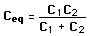so the resonant frequency is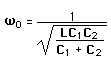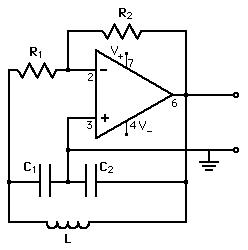For component values

Capacitor C1 = mF
Capacitor C2 = mF
Inductor L = mH

the frequency is f = kHz = MHz = x10^ Hz

Calculation notes: If component values are changed, the new frequency will be calculated. If a frequency is entered, then you must click on the active component name of the component you wish to calculate to force the frequency to match the entered value.

 Other Oscillators
Index

Electronics concepts

Op-amp concepts

 HyperPhysics*****Electricity and magnetism R Nave
Go Back